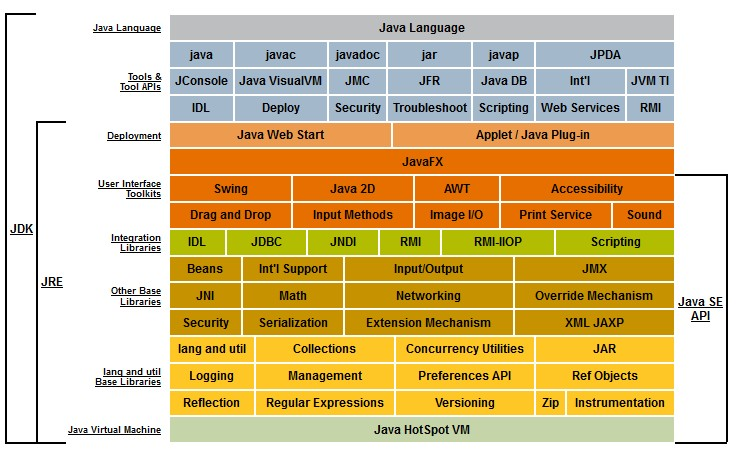# Java - Character (char)

character in java.

There is two type:

They are only manipulated in unicode because the Java platform stores character values using Unicode conventions.

## Management

The Character class wraps a char variable but also provides several management methods such as:

• determining a character's category (lowercase letter, digit, etc.)
• or converting characters from uppercase to lowercase and vice versa.

Character information is based on the Unicode Standard, version 6.0.0 and is based on the data file.

## Syntax

### Init

#### Literal

• A char is quoted in single quote
````char c = '"';`
```

literal notation where the unicode code point (heaxdecimal) starts with a \u. Example with the box drawing character U+2514

````char c = '\u2514';`
```
````((char) Integer.parseInt("2514", 16))`
```

#### Integer (Unicode)

Java uses an int internaly (and not a hexadecimal) to store an unicode character, therefore you can cast the integer represention of the unicode hexadecimal representation to get a character.

Example with the box drawing character U+2514

• 2514 is equivalent to the number 9492, proof:
``````int boxDrawAsInt =  Integer.parseInt("2514", 16);
System.out.println("Decimal Number: "+boxDrawAsInt);```
```
``````Decimal Number: 9492
```
```
• You can cast it directly
``````char boxDrawAsChar = (char) 9492;
System.out.println("Box Draw: "+boxDrawAsChar);```
```
``````Box Draw: └
```
```

### To

#### To String

To string …

````String.valueOf((char) Integer.parseInt("2514", 16))`
```

#### To Int

• for a char
````int i = (int) '"';`
```
• it works also from a character
````int i = (int) ((Character) '"')`
```

#### To Unicode code point

``````char c = '⡎';
System.out.println(Integer.toHexString((int) c));```
```

which leads to the character cldr/utility/character.jsp - braille pattern dot

``````284e
```
```

### Equality

``````char c = '\u0000';
char d = 0;
if (c == d){
System.out.println("They are the same");
}```
```

Because internally a character is an integer, you can do all integer mathematics operations.

````range = (char) this.max - (char) this.min`
```

### Repeat

• General
``````n = 10;
c = 'a'
nc = new String(new char[n]).replace("\0", c);```
```
• From java 11 as the repeat function
````"a".repeat(3);`
```

### Numeric Value

getNumericValue does not return the int decimal representation of Unicode but the real numeric value of the character following this rule:

• 0 to 9 for the digits
• then the characters below have a numeric values from 10 through 35.
• The letters A-Z in their uppercase ('\u0041' through '\u005A'),
• lowercase ('\u0061' through '\u007A'),
• and full width variant ('\uFF21' through '\uFF3A' and '\uFF41' through '\uFF5A')

Snippet:

``````if (Character.isDigit(x)) {
int y = Character.getNumericValue(x);
System.out.println(y);
}```
```

### Array

#### Contains

``````char[] endOfLineCharacters = {(char) 10, (char) 13};
String.valueOf(endOfLineCharacters).contains(String.valueOf((char) 10)));```
```
``````boolean contains(char[] charArray, char c){
boolean contains = false;
for (char cA : charArray) {
if (cA == c) {
contains = true;
break;
}
}
return contains;
}```
```

## Documentation / Reference

Recommended PagesJava - (Primitive|Native) Data Type

Java has a fixed set of primitive types: boolean, byte, short, int, long, char, float, and double. They can also be used in a wrapper class in order to enhance the functionalities....Java - IO - Character Stream

Character streams are build above byte stream. They decodes bytes into characters (or the other way around) using a specified charset. The Java platform stores character values using Unicode conventions....Java - Variable Memory Size

memory size of a variable. To get the memory size of a variable, you can do it: statically with the data type declaration dynamically with the Java runtime. See A single alphanumeric character...Java strings - null terminated

Java strings are not terminated with a null characters as in C or C++. However, when working with Jdbc, you can get Java String that are Null terminated. Null-terminated_string